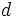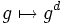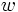Degree homomorphism of a compact connected Lie group

Let$G$ be a compact connected Lie group. Then the degree homomorphism from$G$ to$G$ is a homomorphism of multiplicative monoids:$\mathbb{Z} \to \mathbb{Z}$
that sends an integer$d$ to the degree of the map$g \mapsto g^d$.
The degree homomorphism can be used to compute the degree of any map from$G$ to$G$ defined by a word. This is because if$w(x)$ is a word involving an indeterminate$x$, then all the letters of$w$ other than$x$ or$x^{-1}$, can be homotoped to the identity element.